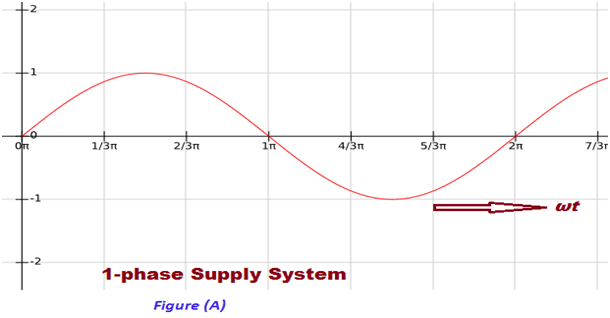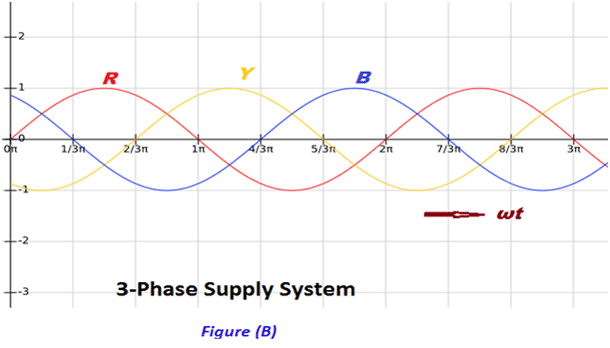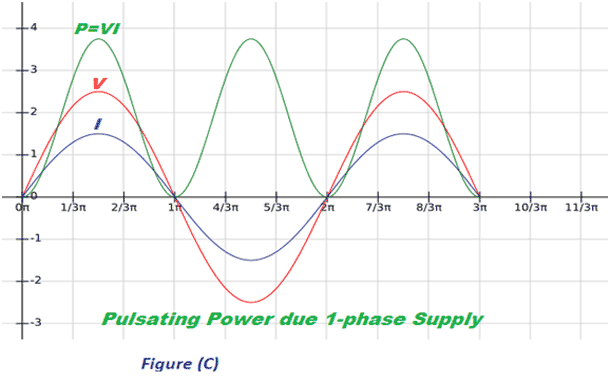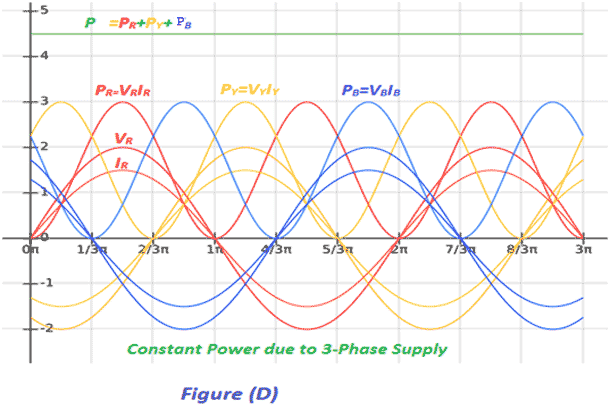# Advantages of Three Phase System over Single Phase System

Presently 3-ø AC system is very popular and being used worldwide for power generation, power transmission, distribution and for electric motors.Three phase system has the following advantages as compare to single phase system:

1. Power to weight ratio of 3-ø alternator is high as compared to 1-ø alternator. That means for generation for same amount of Electric Power, the size of 3-ø alternator is small as compare to 1-ø alternator. Hence, the overall cost of alternator is reduced for generation of same amount of power. Moreover, due to reduction in weight, transportation and installation of alternator become convenient and less space is required to accommodate the alternator in power houses.
2. For electric power transmission and distribution of same amount of power, the requirement of conductor material is less in 3-ø system as compare to 1-ø system. Hence, the 3-ø transmission and distribution system is economical as compare 1-ø system.
3. Let us consider the power produced by single phase supply and 3-phase supply at unity power factor. Waveform of power produce due 1-phase supply at unity power factor is shown in figure (C), and figure (D) shows the waveform of power produced due to 3-phase supply.
4.5. From power waveforms shown in figure (C) and (D) above it is clear that in the 3-phase system, the instantaneous power is almost constant over the cycle results in smooth and vibration-free operation of the machine. Whereas in 1-ø system the instantaneous power is pulsating hence change over the cycle, which leads to vibrations in machines.
6. Power to weight ratio of three phase induction motor is high as compare to single phase induction motor. Means for the same amount of Mechanical Power, the size of three phase induction motor is small as compared to single phase induction motor. Hence, the overall cost of induction motor is reduced. Moreover, due to reduction in weight, transportation, and installation of induction motor become convenient and less space is required to accommodate the induction motor.
7. 3-phase induction motor is self-started as the magnetic flux produced by 3-phase supply is rotating in nature with a constant magnitude. Whereas 1-ø induction motor is not self-started as the magnetic flux produced by 1-ø supply is pulsating in nature. Hence, we have to make some arrangement to make the 1-ø induction motor self-started which further increases the cost of 1-ø induction motor.
8. 3-phase motor is having better power factor
9. Power to weight ratio of 3-phase transformer is high as compare to 1-ø transformer. Means for the same amount of Electric Power, the size of 3-phase transformer is small as compared to 1-ø transformer. Hence, the overall cost of a transformer gets reduced. Moreover, due to reduction in weight, transportation and installation of transformer become convenient and less space is required to accommodate the transformer.
10. If a fault occurs in any winding of a 3-phase transformer, the rest of two winding can be used in the open delta to serve the 3-phase load. The same is not possible in 1-ø transformer. This ability of 3-phase transformer further increases the reliability of 3-phase transformer.
11. A 3-phase system can be used to feed a 1-ø load, whereas vice-versa is not possible.
12. DC rectified from 3-phase supply is having the ripple factor 4% and DC rectified from 1-ø supply is having the ripple factor 48.2%. Mean DC rectified from 3-ø supply contains fewer ripples as compare to DC rectified from a 1-ø supply. Hence the requirement of the filter is reduced for DC rectified from a 3-phase supply. Which reduce the overall cost of converter.

From above it is clear the 3-phase system is more economical, efficient, reliable and convenient as compared to 1-ø system.

Want To Learn Faster? 🎓
Get electrical articles delivered to your inbox every week.
No credit card required—it’s 100% free.